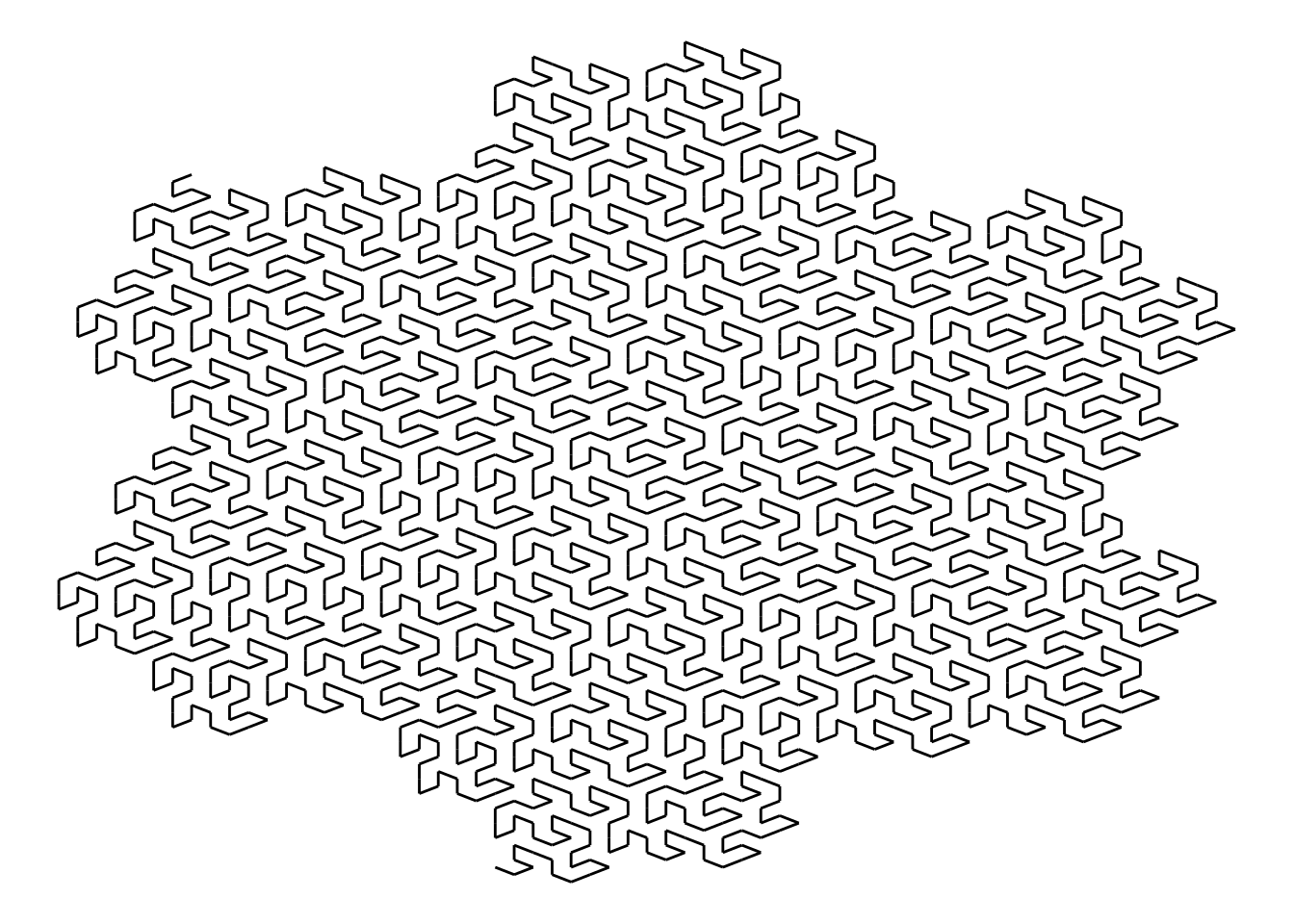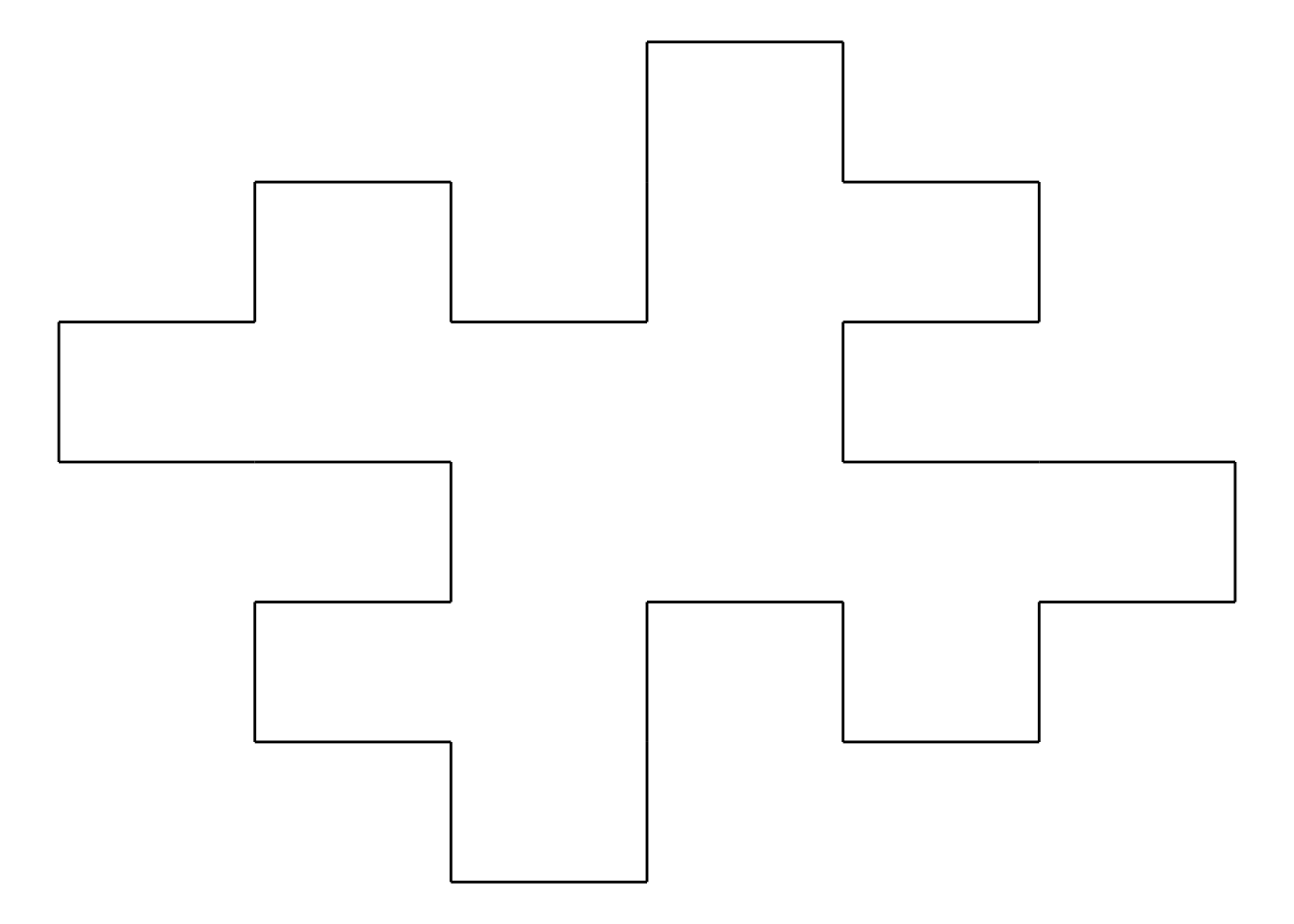# Fractals: using R, R6 classes and recursion.Hexagonal Gosper curve.

I am a fan of fractals and recently I wanted to learn more about object-oriented programming in R using classes. Adam Spannbauer has an excellent tutorial using R6 classes and ggplot2 to create fractal trees and I adapted it for L-system line fractals found in Przemyslaw Prusinkiewicz and Aristid Lindenmayer’s book The Algorithmic Beauty of Plants.

An example of an L-system line fractal follows.

Imagine you are a turtle drawing a line according to instructions: + means turn to the right by 90 degrees. – means turn left 90 degrees. F means move forward a set distance. (Let’s assume it’s 100 pixels.)

So F-F-F-F would draw a square.

F also has a special role in that it replicates itself as a “generator”. If the generator for F is F-F+F+FF-F-F+F then the F-F-F-F would become F-F+F+FF-F-F+F-F-F+F+FF-F-F+F-F-F+F+FF-F-F+F-F-F+F+FF-F-F+F and be drawn like the shape below. Recursion is used to generate subsequent generations of the same repeating pattern which can be seen here.Koch island, generation 1.

Images of more fractals are on these pages. This project also allowed me to try Github pages and Binder. The repository for the code used is here: https://github.com/jeffblackadar/fractal.

Posted on